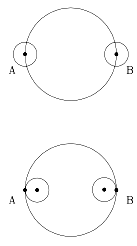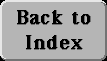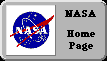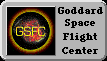# (8c) The Moon's Distance--2

Hipparchus (and possibly Aristarchus before him) also derived the Moon's distance from the duration of a lunar eclipse.

Because the Earth casts a much larger shadow than the Moon, such an eclipse usually darkens the entire Moon, for several hours. Let t be the length of the eclipse, from the moment the shadow touches the middle of the Moon to the moment it again uncovers it.

If the Sun is assumed to be very distant, and if the Moon crosses the middle of the shadow (if not, corrections must be made), the width of the shadow is expected to be about 2r, twice the Earth's radius r.

Let T be the period of the Moon's motion, in a frame rotating with the Earth around the Sun (new Moon to new Moon). Then if R is the Moon's distance, the path covered by the Moon in that period (in the rotating frame) is (2pR) where p = 3.1415926.... It follows that

(2pR)/ 2r = T/ t

and that gives the ratio R/ r, the Moon's distance expressed in Earth radii.Actually, a correction is needed before that equation can be applied. If the Sun were point-like, very small, then a point on the Moon would enter the shadow when point A on Earth covered the center of the Sun, and would end when point B uncovered it (top drawing).

In fact, complete darkness only begins after point A has covered the last bit of the Sun, which happens when the center is one solar radius (apparent radius, as seen from Earth) inside point A. It ends when the first bit of the Sun extends past B, i.e. when the center is one solar radius short of B (bottom drawing).

As a result, to obtain a full width of 2r, the width of the apparent diameter of the Sun must be added to the width of the observed shadow. Since the Moon's apparent size is about the same as the Sun's (= 0.5 degrees), that result is also obtained by timing the interval t from "first contact" when the full shadow first hits the edge of the Moon, to "last contact" when it completely clears the Moon.

Further Exploring:    #8d  Parallax

Next Stop:    #9  The Discovery of the Solar SystemAuthor and curator: David P. Stern, u5dps@lepvax.gsfc.nasa.gov
Last updated 4 February 1999 Moon.